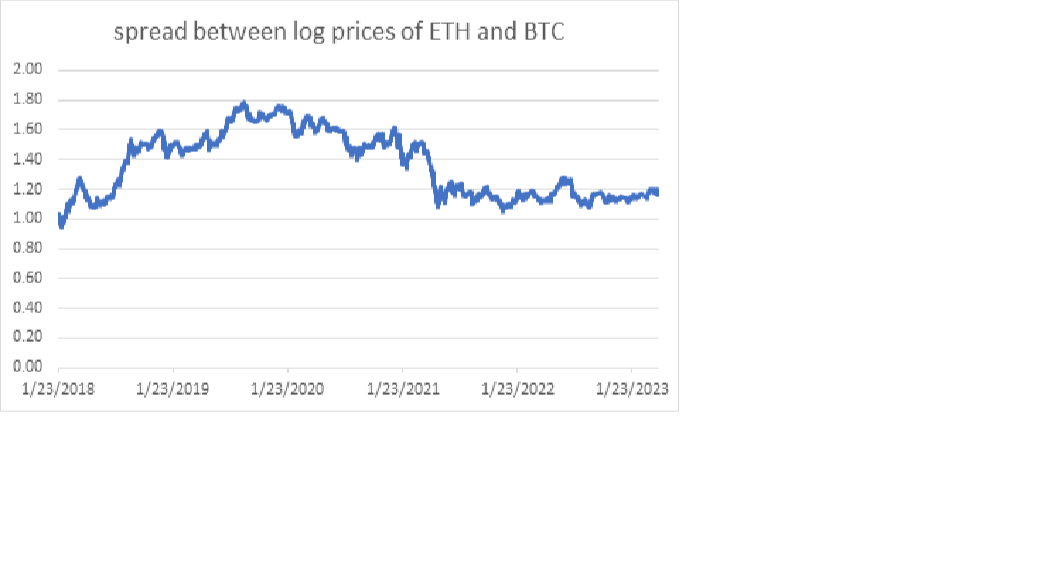Pairs trading is a market-neutral, non-directional strategy seeking to exploit the mispricing between correlated assets. As such, pairs trading involves taking a long and a short position simultaneously in two securities with a high correlation. Suppose there are two securities that have been observed to be correlated. You measure the long-run spread between these co-moving assets. If the spread starts to diverge from the historical spread value, you buy the undervalued security while shorting the overvalued one.

An example will clarify the concept. Let’s look at the two most popular cryptocurrencies, BTC and ETH. Since BTC price is 10x that of ETH, we can take their logs to make it “apples-to-apples” comparison.

The correlation between these two assets is 0.92 which means that these two digital assets are co-moving.

Now let’s take a look at the spread between these log prices.

We see that beginning from roughly May 2021, the spread trades between 1.05 and 1.2 with the mean value of 1.15. In 2022 June the spread diverged from its range rising to 1.28. But then it reverted to its mean quickly. When the spread broke the upper bound of the range at 1.20 and increased to 1.28 on 2022 June 22, you could buy the undervalued ETH and short the overvalued BTC at the same time. The spread reverted to its mean a month later. With the power of hindsight, if you did this trade, it would be profitable. During this time period, prices of both assets rose but ETH jumped 50% while BTC rose “only” 13%. So, the gain from the ETH leg of the trade would not only compensate loss from the short BTC trade but would deliver handsome profit.

So, the basic framework for pairs trading for cryptocurrencies is as follows. First, we take a universe of cryptocurrencies. Then, we look at pairwise relationships of the tokens in our universe. We select the top, say, 20 pairs to trade. When the spread in one of these pairs diverges from its mean during the training period, we open a trade buying a cheap token while selling an expensive one at the same time.fmiren